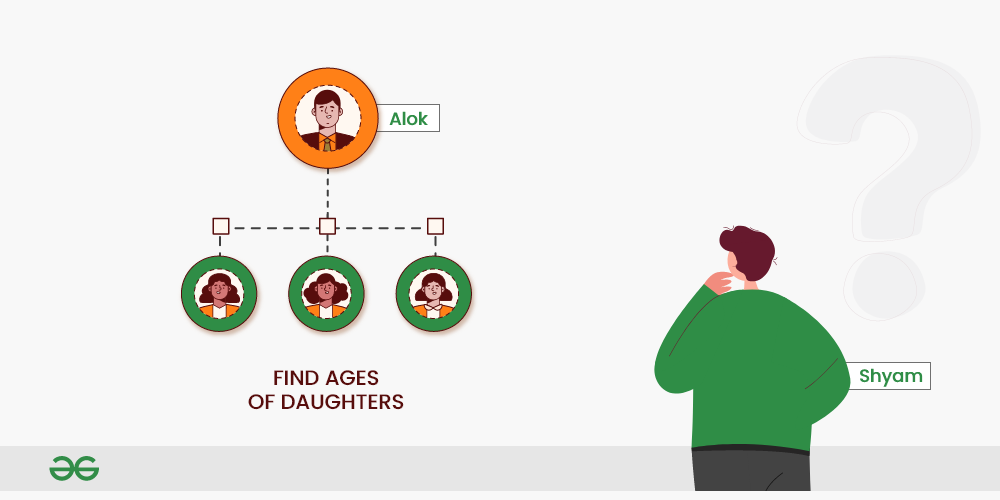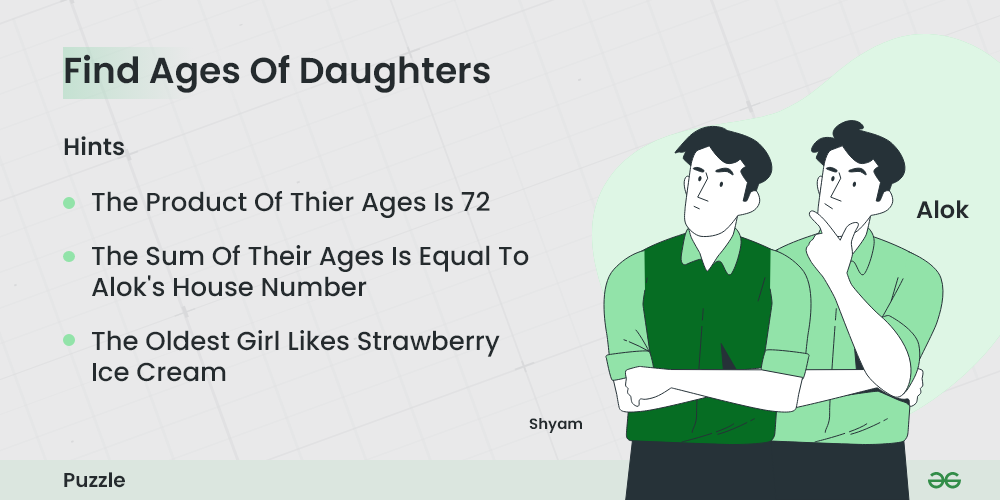# Puzzle 2 | (Find the ages of daughters)

Alok has three daughters. His friend Shyam wants to know the ages of his daughters. Alok gives him a first hint.Find the ages of daughters

1. The product of their age is 72.

Shyam says this is not enough information Alok gives him a second hint.

2. The sum of their ages is equal to my house number.

Shyam goes out and looks at the house number and tells “I still do not have enough information to determine the ages”.

Alok admits that Shyam can not guess and gives him the third hint

3. The oldest girl likes strawberry ice cream.

Shyam is able to guess after the third hint. Can you guess what are the ages of the three daughters?Find the ages of daughters

1. Product of ages is 72

Below are all possibilities to get 72 from product of three different ages:

1 * 1 * 72 = 72

1 * 2 * 36 = 72

1 * 3 * 24 = 72

1 * 4 * 18 = 72

1 * 6 * 12 = 72

1 * 8 * 9 = 72

2 * 2 * 18 = 72

2 * 3 * 12 = 72

2 * 4 * 9 = 72

2 * 6 * 6 = 72

3 * 3 * 8 = 72

3 * 4 * 6 = 72

2. Sum of the ages is given

1 + 1 + 72 = 74

1 + 2 + 36 = 39

1 + 3 + 24 = 28

1 + 4 + 18 = 23

1 + 6 + 12 = 19

1 + 8 + 9 = 18

2 + 2 + 18 = 22

2 + 3 + 12 = 17

2 + 4 + 9 = 15

2 + 6 + 6 = 14

3 + 3 + 8 = 14

3 + 4 + 6 = 13

All sums are unique except 14. So the age sum must have been 14, otherwise, Shyam would have guessed the ages from hint 2 only.

So we have two possible combinations to get a sum of 14

2 + 6 + 6 = 14

3 + 3 + 8 = 14

3.  Alok has the oldest girl (not two!!). So the ages must be 3, 3 and 8.

Whether you're preparing for your first job interview or aiming to upskill in this ever-evolving tech landscape, GeeksforGeeks Courses are your key to success. We provide top-quality content at affordable prices, all geared towards accelerating your growth in a time-bound manner. Join the millions we've already empowered, and we're here to do the same for you. Don't miss out - check it out now!

Previous
Next